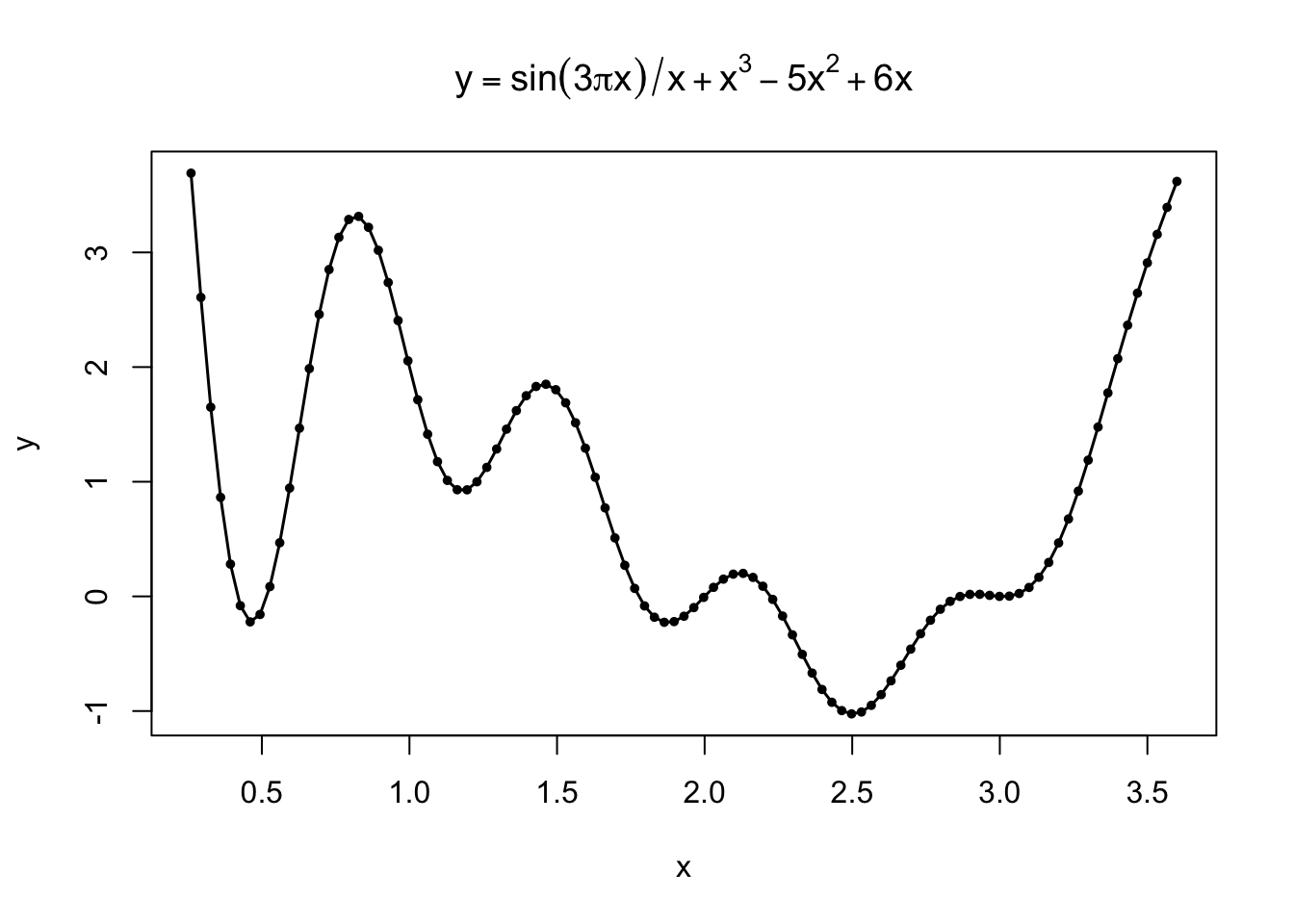# Introduction

Bayesian optimisation (BO) is a sequential sampling technique for global optimisation. It has two appealing features: ability to handle complex objective functions and sample efficiency. The basic machinery of BO consists of:

• Gaussian process surrogate models for estimating objective functions, and
• Acquisition function for prescribing the next sampling location.

These two components dynamically interact with each other to achieve sample efficient global optimisation. Consequently, BO is particularly useful when function evaluations are expensive and the gradient information is not available.

This sequential “sampling technique” is different from Monte Carlo sampling methods, which are about how to sample from specific distributions. Here, some stochastic process is given, and we are assumed to have access to a sampler for that stochastic process no matter what input we use. The problem is what input from the index set to use. Imagine a noisy objective function, which is modelled as a stochastic process. In other words, the problem is where to evaluate the objective function.

## Global optimisation

Recall from the optimisation notes, global optimisation can be formulated as solving the following problem $\min_{x\in\mathcal{D}} f(x)$ where $$f$$ is the objective function and $$\mathcal{D}$$ is the choice set in which we try to find a minimiser $$x^*$$ such that $$f(x^*)\leq f(x)$$ for all $$x\in\mathcal{D}$$.

In these notes, we use minimisation to formulate optimisation problems.

The fundamental challenge arises from our limited knowledge of the shape of $$f$$. Unless $$f$$ is fairly simple such as low-degree polynomials and sinusoids, it is usually hard to get a global picture from the expression of $$f$$ even in 1-D cases.

Most of the times, therefore, we evaluate $$f$$ at a finite number of points and interpolate in-betweens. Using the same test function as in the optimisation notes, for example, the following graph is produced by first evaluating at 101 evenly spaced points from 0.26 to 3.6 and then connecting dots. The global minimum is approximately $$-1.025$$ at $$x=2.5$$.With this graph, we can easily pick the best one (68th point) among 101 points as an approximate minimiser $$\hat{x}^*=2.498$$ yielding $$f(\hat{x}^*)=-1.025$$, which is essentially the global minimum.

Comparing to this simple yet effective approach, we notice something naïve in gradient descent; it does not utilise information that can be extracted from visited/evaluated points. Instead, it ignores the history but uses only local information (i.e., gradient) and blindly follows the direction at each step. As a result, if using random initial points to find the global minimum, we must repeat the process at least five times (very lucky) in the above case

## Surrogate models

Seeing those evaluated points as data, don’t you think the above interpolation is akin to statistical regression? In regression, we collect data $$(X,\mathbf{y})$$ and estimate the underlying data-generating process by fitting some models to the data. Above, using the evenly-spaced points $$X$$ and the corresponding objective values $$\mathbf{y}=f(X)$$, we essentially estimate the underlying objective function by connecting dots.

When we do regression, our models are more sophisticated than connecting data points (e.g., linear, polynomial, neural network, etc.). So, why not using statistical models for optimisation as well? It is reasonable to expect that suitable models could extract more information from evaluated points about the objective function and, in particular, promising regions of minimisers, leading to more efficient search. These models are commonly called surrogates.

Connecting dots can be seen as a special case of spline or local regression.

# Gaussian process

## Definition

Gaussian process (GP) is a collection of random variables (i.e., stochastic process) such that any finite sub-collection follows a multivariate normal distribution. GP is the most popular surrogate models in Bayesian optimisation.

Remember the Poisson process is a stochastic process specified by rate $$\lambda$$. For a counting process, we use a set of nonnegative real numbers $$\mathbb{R}_+$$ as an index set, with which for some epoch $$t\in\mathbb{R}_+$$ of interest, we have a counting random variable $$N(t)\sim\text{pois}(\lambda t)$$.

You may also find GP classified as Bayesian nonparametric in statistics and machine learning.

To satisfy the above condition, a GP is specified by a mean function $$m$$ and covariance function $$k$$. We write $$Y\sim\mathcal{GP}(m,k)$$ to denote that a stochastic process $$Y$$ follows a GP specified by $$m$$ and $$k$$. As the names suggest, the roles of $$m$$ and $$k$$ are as follows. For inputs $$x$$ and $$x'$$ of the index set, \begin{align} m(x) &= \mathbb{E}[Y(x)]\\ k(x,x') &= \mathbb{E}[(Y(x)-m(x))(Y(x')-m(x'))]. \end{align}

Intuitively, $$k(x,x')$$ measures “distance” between $$x$$ and $$x'$$ reflecting similarity between $$Y(x)$$ and $$Y(x')$$. If two inputs $$x$$ and $$x'$$ are close, the corresponding random variables $$Y(x)$$ and $$Y(x')$$ should be strongly correlated.

For concreteness, suppose the index set is the real line $$\mathbb{R}$$. Then, if we are interested in a collection of two random variables at $$\mathbf{x}=(x_1,x_2)$$, then a random vector $Y = \begin{bmatrix} Y(x_1)\\ Y(x_2) \end{bmatrix}$ is jointly distributed as $$\mathrm{N}(\boldsymbol{\mu},\Sigma)$$ where $\boldsymbol{\mu} = \begin{bmatrix} m(x_1)\\ m(x_2) \end{bmatrix} \quad\text{and}\quad \Sigma = \begin{bmatrix} k(x_1,x_1) & k(x_1,x_2)\\ k(x_2,x_1) & k(x_2,x_2) \end{bmatrix}.$

Remember the property of Normal distribution. To have the joint PDF, in practice, we define $$k$$ such that $$\Sigma$$ is positive definite.

### Demo

Let’s see what GP realisation looks like, using one of the most basic configurations: $$m(x) = 0$$ and $$k(x,x') = \exp(-\|x-x'\|^2)$$.

k <- function(x1,x2) exp(-(x1-x2)^2)

xx <- seq(.26, 3.6, length.out=101)
K <- outer(xx, xx, k) # 101×101 covariance matrix
Y <- mvrnorm(3, rep(0,m), K)
matplot(xx, t(Y), type="o", lwd=1.2, pch=20, cex=0.5, xlab="x", ylab="y")
title("Random functions from GP")